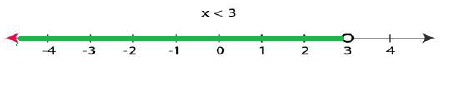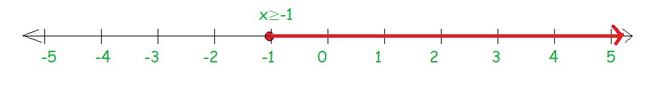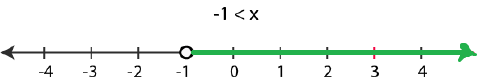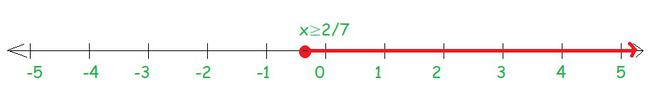GFG App
Open AppBrowser
Continue

# Class 11 NCERT Solutions – Chapter 6 Linear Inequalities – Exercise 6.1 | Set 2

### Question 13. 2 (2x + 3) – 10 < 6 (x – 2)

Solution:

Given, 2(2x + 3) – 10 < 6 (x – 2)
By multiplying we get
4x + 6 – 10 < 6x – 12
On simplifying we get
4x – 4 < 6x – 12
– 4 + 12 < 6x – 4x
8 < 2x
4 < x
Thus, the solutions of the given equation are defined by all the real numbers greater than or equal to 4.
Hence, the required solution set is [4, ∞)

### Question 14. 37 – (3x + 5) ≥ 9x – 8 (x – 3)

Solution:

Given that, 37 – (3x + 5) ≥ 9x – 8 (x – 3)
On simplifying we get
37 – 3x – 5 ≥ 9x – 8x + 24
32 – 3x ≥ x + 24
On rearranging
32 – 24 ≥ x + 3x
8 ≥ 4x
2 ≥ x
All the real numbers of x which are less than or equal to 2 are the solutions of the given equation
Hence, (-∞, 2] will be the solution for the given equation

### Question 15. x/4<(5x-2)/3-(7x-3)/5

Solution:

Given, x/4<(5x-2)/3-(7x-3)/5
Taking 15 as the lcm on the right side
x/4<(5(5x-2)-3(7x-3))/15
Further, simplifying we get,
x/4<((25x-10)-(21x-9))/15
Multiplying 15 at both the sides
15x/4<25x-10-21x+9
15x/4<4x-1
Multiplying by 4 we get
15x < 4 (4x – 1)
15x < 16x – 4
4 < x
All the real numbers of x which are greater than 4 are the solutions of the given equation
Hence, (4, ∞) will be the solution for the given equation

### Question 16. (2x-1)/3 >= (3x-2)/4-(2-x)/5

Solution:

Let us simplify the inequation
(2x-1)/3>=(5(3x-2)-4(2-x))/20
(2x-1)/3>=((15x-10)-(8-4x))/20
(2x-1)/3>=(15x-10-8+4x)/20
(2x-1)/3>=(19x-18)/20
Now cross multiplying at both the sides we get
20 (2x – 1) ≥ 3 (19x – 18)
40x – 20 ≥ 57x – 54
– 20 + 54 ≥57x – 40x
34 ≥ 17x
2 ≥ x
∴ All the real numbers of x which are less than or equal to 2 are the solutions of the given equation
Hence, (-∞, 2] will be the solution for the given equation

### Question 17. 3x – 2 < 2x + 1

Solution:

Given, 3x – 2 < 2x + 1Solving the given inequality, we get
3x – 2 < 2x + 1
3x – 2x < 1 + 2
x < 3
Now, the graphical representation of the solution is as follows:### Question 18. 5x – 3 ≥ 3x – 5

Solution:

We have,5x – 3 ≥ 3x – 5
Solving the given inequality, we get
5x – 3 ≥ 3x – 5
On rearranging we get
5x – 3x ≥ -5 + 3
On simplifying 2x ≥ -2
Now divide by 2 on both sides we get
x ≥ -1
The graphical representation of the solution is as follows:### Question 19. 3 (1 – x) < 2 (x + 4)

Solution:

Given,3 (1 – x) < 2 (x + 4)
Solving the given inequality, we get
3 (1 – x) < 2 (x + 4)
Multiplying we get 3 – 3x < 2x + 8
On rearranging we get
3 – 8 < 2x + 3x
– 5 < 5x
Now by dividing 5 on both sides we get
-5/5 < 5x/5
– 1 < x
Now, the graphical representation of the solution is as follows:### Question 20. x/2>=(5x-2)/3-(7x-3)/5

Solution:

Let us solve the right side of the inequation
x/2>=(5(5x-2)-(7x-3)3)/15
x/2>=(25x-10-21x+9)/15
x/2>=(4x+1)/15
15x ≥ 2 (4x – 1)
15x ≥ 8x -2
15x -8x ≥ 8x -2 -8x
7x ≥ -2
x ≥ -2/7

Now, the graphical representation of the solution is as follows:### Question 21. Ravi obtained 70 and 75 marks in first two unit test. Find the minimum marks he should get in the third test to have an average of at least 60 marks.

Solution:

Let us assume that the marks obtained by Ravi in his third unit test be x,
According to question,
the entire students should have an average of at least 60 marks i.e,
(70 + 75 + x)/3 ≥ 60
145 + x ≥ 180
x ≥ 180 – 145
x ≥ 35

Thus, all the students must obtain 35 marks in order to have an average of at least 60 marks

### Question 22. To receive Grade ‘A’ in a course, one must obtain an average of 90 marks or more in five examinations (each of 100 marks). If Sunita’s marks in first four examinations are 87, 92, 94 and 95, find minimum marks that Sunita must obtain in fifth examination to get grade ‘A’ in the course.

Solution:

Let us assume Sunita scored x marks in her 5th examination
Now, according to the question
In order to receive A grade in the course she must have to obtain average 90 marks or more in her five examinations that is,
(87 + 92 + 94 + 95 + x)/5 ≥ 90
(368 + x)/5 ≥ 90
368 + x ≥ 450
x ≥ 450 – 368
x ≥ 82
Thus, she must have to obtain 82 or more marks in her fifth examination

### Question 23. Find all pairs of consecutive odd positive integers both of which are smaller than 10 such that their sum is more than 11.

Solution:

Let us assume x be the smaller of the two consecutive odd positive integers
Let other integer = x + 2
It is also given in the question that,
both the integers are smaller than 10 that is,
x + 2 < 10

x< 8 … (a)
Also, it is given in the question that sum of two integers is more than 11
∴ x + (x + 2) > 11
2x + 2 > 11
x > 9/2
x > 4.5 … (b)
Thus, from (a) and (b) we have x is an odd integer and it can take values 5 and 7
Hence, possible pairs are (5, 7) and (7, 9)

### Question 24. Find all pairs of consecutive even positive integers, both of which are larger than 5 such that their sum is less than 23.

Solution:

Let us assume x be the smaller of the two consecutive even positive integers
Let other integer= x + 2
It is also given in the question that, both the integers are larger than 5∴
x > 5   ….(a)
Also, it is given in the question that the sum of two integers is less than 23.
∴ x + (x + 2) < 23
2x + 2 < 23
x < 21/2
x < 10.5   …. (b)
Thus, from (a and (b) we have x is an even number and it can take values 6, 8 and 10

Thus, the possible pairs are (6, 8), (8, 10) and (10, 12).

### Question 25. The longest side of a triangle is 3 times the shortest side and the third side is 2 cm shorter than the longest side. If the perimeter of the triangle is at least 61 cm, find the minimum length of the shortest side.

Solution:

Let us assume that the length of the shortest side of the triangle be x cm
According to the question,
length of the longest side = 3x cm
And, length of third side = (3x – 2) cm
As, the least perimeter of the triangle = 61 cm
Thus, x + 3x + (3x – 2) cm ≥ 61 cm
7x – 2 ≥ 61
7x ≥ 63
Now let us divide by 7 we get=
7x/7 ≥ 63/7=
x ≥ 9

Hence, the minimum length of the shortest side will be 9 cm

### Question 26. A man wants to cut three lengths from a single piece of board of length 91cm. The second length is to be 3cm longer than the shortest and the third length is to be twice as long as the shortest. What are the possible lengths of the shortest board if the third piece is to be at least 5cm longer than the second?

Solution:

Let us assume that the length of the shortest piece be x cm
∴ According to the question,
Length of the second piece = (x + 3) cm
And, length of third piece = 2x cm
As all the three lengths are to be cut from a single piece of board having a length of 91 cm
x + (x + 3) + 2x ≤ 91 cm
4x + 3 ≤ 91
4x ≤ 88
4x/4 ≤ 88/4
x ≤ 22 … (i)
Also, it is given in the question that, the third piece is at least 5 cm longer than the second piece
2x ≥ (x+3) + 5
2x ≥ x + 8
x ≥ 8 … (ii)
Thus, from equation (i) and (ii) we have:
8 ≤ x ≤ 22
Hence, it is clear that the length of the shortest board is greater than or equal to 8 cm and less than or equal to 22 cm

My Personal Notes arrow_drop_up